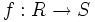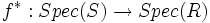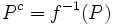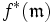# Integral extension implies inverse image of max-spectrum is max-spectrum

This article gives the statement, and possibly proof, of a fact about how a property of a homomorphism of commutative unital rings, forces a property for the induced map on spectra
View other facts about induced maps on spectra

## Statement

Suppose$f:R \to S$ is an integral extension of commutative unital rings. Then consider the induced map on spectra:$f^*: Spec(S) \to Spec(R)$

that sends a prime ideal$P$ of$S$ to its contraction$P^c = f^{-1}(P)$ in$R$.

Then the following are true:

• If$\mathfrak{m}$ is a maximal ideal of$S$, then$f^*(\mathfrak{m})$ is maximal in$R$
• If$f^*(P)$ is maximal in$R$, then$P$ is maximal in$S$

## Proof

The key idea here is the following fact: for a finite extension of integral domains, one of them is a field if and only if the other is.